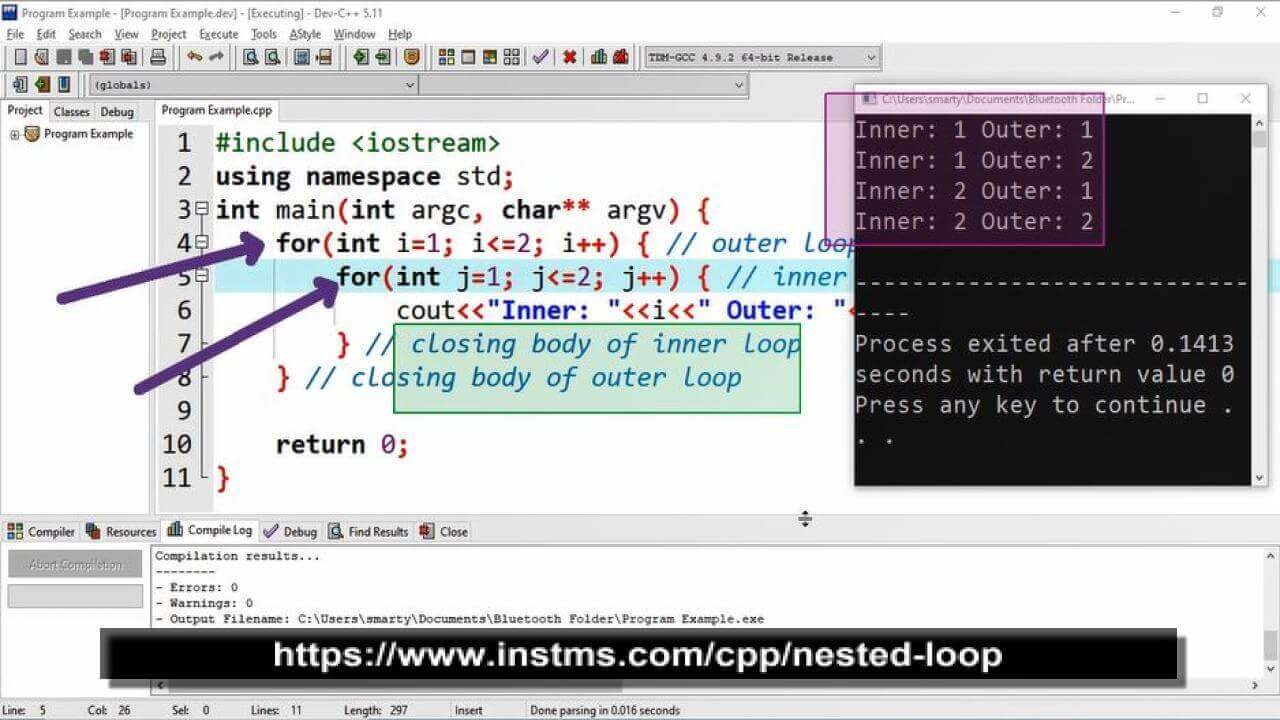# Nested loop in C++

Loop containing other loops inside its body is called nested loop. Nested loop may have multiple levels. Commonly it is used upto two level. Any one out of while, do while and for loop can be used as inner and outer loop. To make shorter structure of nested loop we can use for loop.

Nested loop structure is commonly used for the following purposes.

• Matrix Arrays Manipulation
• Complex Computations
• Printing Complex Patterns
• Prcoessing Data Structures

## Syntax

while ( condition ) {

while ( condition ) {

statements;

}

}

## Example

``````#include <iostream>
using namespace std;

int main(int argc, char** argv) {

int m , n ;
m = 1 ;
n = 1 ;

while ( m <= 5 ) {
while ( n <= 5 ) {
cout<<"Outter : "<<m<<" , Inner : "<<n<<endl;
n = n + 1 ;
}
m = m + 1 ;
}

return 0;
}``````

## Output